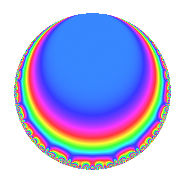# Properties

 Label 6048.2.a.beLevel 6048 Weight 2 Character orbit 6048.a Self dual Yes Analytic conductor 48.294 Analytic rank 0 Dimension 2 CM No Inner twists 1

# Related objects

## Newspace parameters

 Level: $$N$$ = $$6048 = 2^{5} \cdot 3^{3} \cdot 7$$ Weight: $$k$$ = $$2$$ Character orbit: $$[\chi]$$ = 6048.a (trivial)

## Newform invariants

 Self dual: Yes Analytic conductor: $$48.2935231425$$ Analytic rank: $$0$$ Dimension: $$2$$ Coefficient field: $$\Q(\sqrt{17})$$ Coefficient ring: $$\Z[a_1, \ldots, a_{5}]$$ Coefficient ring index: $$1$$ Fricke sign: $$-1$$ Sato-Tate group: $\mathrm{SU}(2)$

## $q$-expansion

Coefficients of the $$q$$-expansion are expressed in terms of $$\beta = \frac{1}{2}(1 + \sqrt{17})$$. We also show the integral $$q$$-expansion of the trace form.

 $$f(q)$$ $$=$$ $$q + \beta q^{5} - q^{7} +O(q^{10})$$ $$q + \beta q^{5} - q^{7} + 2 \beta q^{11} + ( -5 + \beta ) q^{13} + ( -1 + 2 \beta ) q^{17} + ( -2 + 2 \beta ) q^{19} + ( 1 + 3 \beta ) q^{23} + ( -1 + \beta ) q^{25} + ( 7 + \beta ) q^{29} + ( -1 + \beta ) q^{31} -\beta q^{35} + ( 2 - \beta ) q^{37} + ( -10 + \beta ) q^{41} + ( 3 - 4 \beta ) q^{43} + ( -2 + \beta ) q^{47} + q^{49} + ( 1 - 3 \beta ) q^{53} + ( 8 + 2 \beta ) q^{55} + ( -3 - 2 \beta ) q^{59} + ( 2 - 4 \beta ) q^{61} + ( 4 - 4 \beta ) q^{65} + ( 11 - 3 \beta ) q^{67} + ( -1 + \beta ) q^{71} + ( -2 - 2 \beta ) q^{73} -2 \beta q^{77} + ( 6 + \beta ) q^{79} + \beta q^{83} + ( 8 + \beta ) q^{85} + ( -9 + 3 \beta ) q^{89} + ( 5 - \beta ) q^{91} + 8 q^{95} + ( -12 + 4 \beta ) q^{97} +O(q^{100})$$ $$\operatorname{Tr}(f)(q)$$ $$=$$ $$2q + q^{5} - 2q^{7} + O(q^{10})$$ $$2q + q^{5} - 2q^{7} + 2q^{11} - 9q^{13} - 2q^{19} + 5q^{23} - q^{25} + 15q^{29} - q^{31} - q^{35} + 3q^{37} - 19q^{41} + 2q^{43} - 3q^{47} + 2q^{49} - q^{53} + 18q^{55} - 8q^{59} + 4q^{65} + 19q^{67} - q^{71} - 6q^{73} - 2q^{77} + 13q^{79} + q^{83} + 17q^{85} - 15q^{89} + 9q^{91} + 16q^{95} - 20q^{97} + O(q^{100})$$

## Embeddings

For each embedding $$\iota_m$$ of the coefficient field, the values $$\iota_m(a_n)$$ are shown below.

For more information on an embedded modular form you can click on its label.

Label $$\iota_m(\nu)$$ $$a_{2}$$ $$a_{3}$$ $$a_{4}$$ $$a_{5}$$ $$a_{6}$$ $$a_{7}$$ $$a_{8}$$ $$a_{9}$$ $$a_{10}$$
1.1
 −1.56155 2.56155
0 0 0 −1.56155 0 −1.00000 0 0 0
1.2 0 0 0 2.56155 0 −1.00000 0 0 0
 $$n$$: e.g. 2-40 or 990-1000 Significant digits: Format: Complex embeddings Normalized embeddings Satake parameters Satake angles

## Inner twists

This newform does not admit any (nontrivial) inner twists.

## Atkin-Lehner signs

$$p$$ Sign
$$2$$ $$1$$
$$3$$ $$-1$$
$$7$$ $$1$$

## Hecke kernels

This newform can be constructed as the intersection of the kernels of the following linear operators acting on $$S_{2}^{\mathrm{new}}(\Gamma_0(6048))$$:

 $$T_{5}^{2} - T_{5} - 4$$ $$T_{11}^{2} - 2 T_{11} - 16$$ $$T_{13}^{2} + 9 T_{13} + 16$$ $$T_{17}^{2} - 17$$